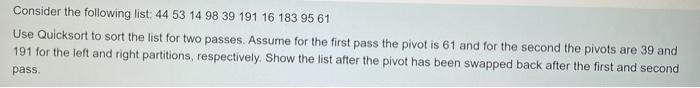# Question Solved1 AnswerConsider the following list: 44 53 14 98 39 191 16 183 95 61 Use Quicksort to sort the list for two passes. Assume for the first pass the pivot is 61 and for the second the pivots are 39 and 191 for the left and right partitions, respectively. Show the list after the pivot has been swapped back after the first and second pass.IBWYYZ The Asker · Computer ScienceTranscribed Image Text: Consider the following list: 44 53 14 98 39 191 16 183 95 61 Use Quicksort to sort the list for two passes. Assume for the first pass the pivot is 61 and for the second the pivots are 39 and 191 for the left and right partitions, respectively. Show the list after the pivot has been swapped back after the first and second pass.
More
Transcribed Image Text: Consider the following list: 44 53 14 98 39 191 16 183 95 61 Use Quicksort to sort the list for two passes. Assume for the first pass the pivot is 61 and for the second the pivots are 39 and 191 for the left and right partitions, respectively. Show the list after the pivot has been swapped back after the first and second pass.